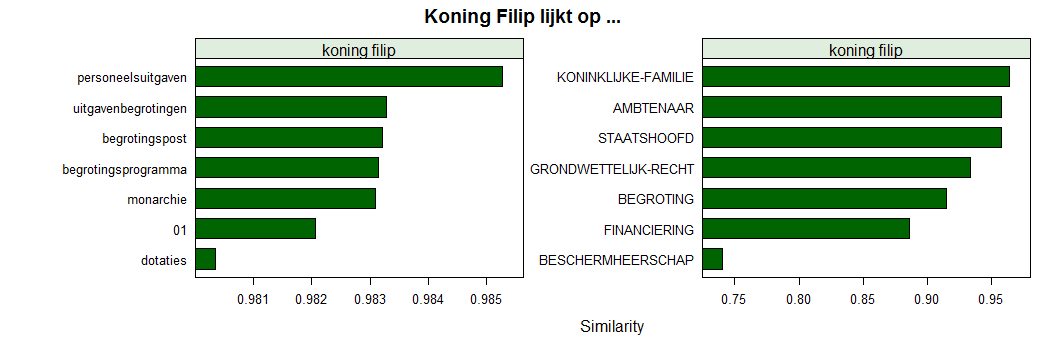# Koning Filip lijkt op ...

Last call for the course on Text Mining with R, held next week in Leuven, Belgium on April 1-2. Viewing the course description as well as subscription can be done at https://lstat.kuleuven.be/training/coursedescriptions/text-mining-with-r

Some things you'll learn ... is that King Filip of Belgium is similar to public expenses if we just look at open data from questions and answers in Belgian parliament (retrieved from here http://data.dekamer.be). Proof is below. See you next week.`library(ruimtehol)library(data.table)library(lattice)library(latticeExtra)data("dekamer", package = "ruimtehol")dekamer\$x <- strsplit(dekamer\$question, "\\W")dekamer\$x <- lapply(dekamer\$x, FUN = function(x) setdiff(x, ""))dekamer\$x <- sapply(dekamer\$x, FUN = function(x) paste(x, collapse = " "))dekamer\$x <- tolower(dekamer\$x)dekamer\$y <- strsplit(dekamer\$question_theme, split = ",")dekamer\$y <- lapply(dekamer\$y, FUN=function(x) gsub(" ", "-", x))set.seed(321)model <- embed_tagspace(x = dekamer\$x, y = dekamer\$y,                         early_stopping = 0.8, validationPatience = 10,                        dim = 50,                         lr = 0.01, epoch = 40, loss = "softmax", adagrad = TRUE,                         similarity = "cosine", negSearchLimit = 50,                        ngrams = 2, minCount = 2)embedding_words  <- as.matrix(model, type = "words")embedding_labels <- as.matrix(model, type = "labels", prefix = FALSE)embedding_person <- starspace_embedding(model, tolower(c("Theo Francken")))embedding_person <- starspace_embedding(model, tolower(c("Koning Filip")))similarities <- embedding_similarity(embedding_person, embedding_words, top = 9)similarities <- subset(similarities, !term2 %in% c("koning", "filip"))similarities\$term <- factor(similarities\$term2, levels = rev(similarities\$term2))plt1 <- barchart(term ~ similarity | term1, data = similarities,          scales = list(x = list(relation = "free"), y = list(relation = "free")),         col = "darkgreen", xlab = "Similarity", main = "Koning Filip lijkt op ...")similarities <- embedding_similarity(embedding_person, embedding_labels, top = 7)similarities\$term <- factor(similarities\$term2, levels = rev(similarities\$term2))plt2 <- barchart(term ~ similarity | term1, data = similarities,          scales = list(x = list(relation = "free"), y = list(relation = "free")),         col = "darkgreen", xlab = "Similarity", main = "Koning Filip lijkt op ...")c(plt1, plt2)`